Home Practice
For learners and parents For teachers and schools
Textbooks
Full catalogue
Pricing SupportLog in

We think you are located in United States. Is this correct?

# Angles formed by lines intersected by a transversal

## 12.4 Angles formed by lines intersected by a transversal

A transversal is a line that crosses at least two other lines.

In the diagram below, the transversals (the blue lines) cut across the black lines.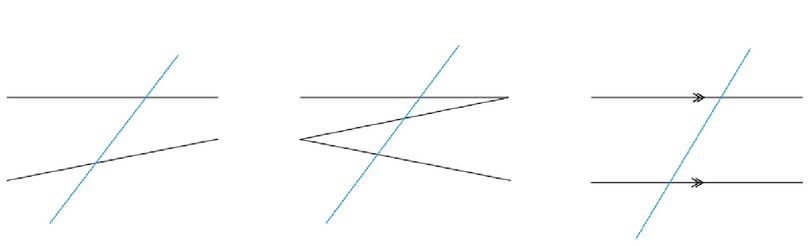transversal
a line that crosses at least two other lines

We will compare the three pairs of angles that are formed when a transversal intersects two lines. These pairs are named by looking at their positions relative to the transversal:

• corresponding angles
• alternate angles
• co-interior angles.

### Corresponding angles

The angles that lie on the same side of the transversal and are in matching positions are called corresponding angles (corresp $$\angle$$s).

corresponding angles
angles that lie on the same side of the transversal and in matching positions
Exercise 12.3 Find corresponding angles

In the diagram, there are four pairs of corresponding angles. Find the corresponding angles.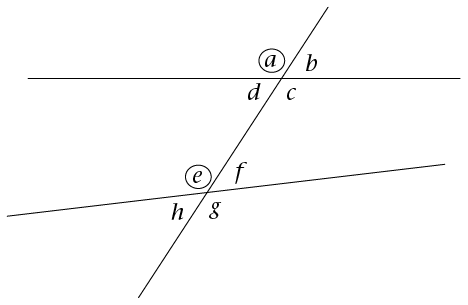• $$a$$ and $$e$$ (they are both left of the transversal and above the lines)
• $$b$$ and $$f$$ (they are both right of the transversal and above the lines)
• $$d$$ and $$h$$ (they are both left of the transversal and below the lines)
• $$c$$ and $$g$$ (they are both right of the transversal and below the lines)

### Alternate angles

Alternate angles (alt $$\angle$$s) lie on opposite (or alternate) sides of the transversal.

alternate angles
angles that lie on opposite (or alternate) sides of the transversal
Exercise 12.4 Find alternate angles

Find the alternate angles in the diagram.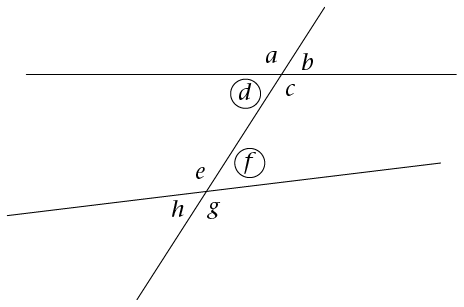In the diagram, these are the alternate angles:

• $$d$$ and $$f$$ (they are on alternate sides of the transversal and lie on the inside of the parallel lines)
• $$c$$ and $$e$$ (they are both on alternate sides of the transversal and lie on the inside of the parallel lines).

### Co-interior angles

Co-interior angles (co-int $$\angle$$s) lie on the same side of the transversal and between the two lines.

co-interior angles
angles that lie on the same side of the transversal and on the inside of the two lines
Exercise 12.5 Find co-interior angles

Find the co-interior angles in the diagram.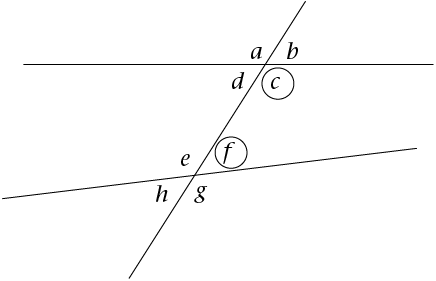In the diagram, these are the co-interior angles:

• $$c$$ and $$f$$ (they are on the same side of the transversal and lie on the insides of both parallel lines)
• $$d$$ and $$e$$ (they are on the same side of the transversal and lie on the insides of both parallel lines).

### Parallel lines intersected by a transversal

Two lines intersect if they cross each other at a point. For example, at a traffic intersection, two or more streets intersect, and the middle of the intersection is the common point between the streets.

Parallel lines are two lines that are a constant distance apart. The symbol $$\parallel$$ is used to indicate parallel lines.

parallel lines
two lines that are a constant distance apart

In this diagram, the lines AB and CD are parallel. We write: $$AB//CD$$.

Parallel lines are indicated by arrow symbols, as shown in the diagram.A transversal line intersects two or more lines. In the diagram above, the line EF cuts AB at G and CD at H. EF is a transversal line.

When parallel lines are cut by a transversal:

• the corresponding angles are equal
• the alternate angles are equal
• the co-interior angles add up to $$180^{\circ}$$.

### Angles formed when parallel lines are cut by a transversal

#### Corresponding angles

Look for angles forming an F pattern. Remember that corresponding angles are the angles in matching positions. They are on the same side of the parallel lines, and on the same side of the transversal. The equal angles are shown in the diagram.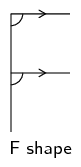If we colour the lines that make corresponding angles, we will see the F shape. This helps us to identify corresponding angles.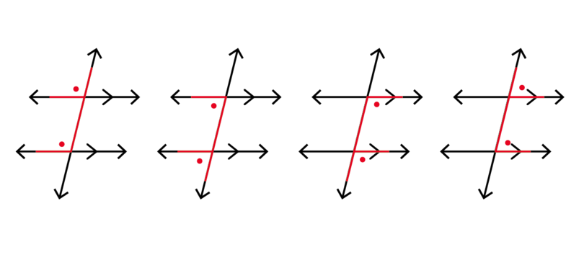#### Alternate angles

Look for angles forming a Z pattern. Remember that alternate angles are the angles that lie on alternate sides of the transversal and inside the parallel lines. Alternate angles are equal.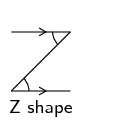If we colour the lines that make alternate angles, we will see a Z shape. This helps us identify alternate angles.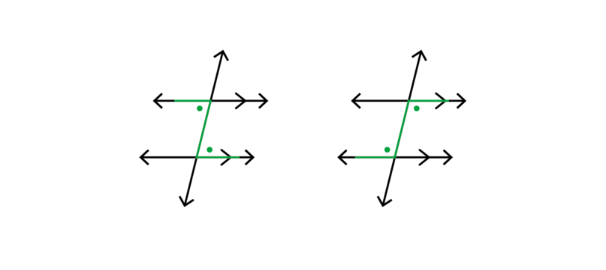#### Co-interior angles

Look for angles forming a C pattern. These are angles that lie in between the parallel lines and on the same side of the transversal. Co-interior angles are supplementary. This means that the sum of these two angles is $$180^{\circ}$$.

Hint: Be careful! These co-interior angles are not equal, but are supplementary.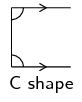If we colour the lines that make co-interior angles, we will see a C shape. This helps us identify co-interior angles.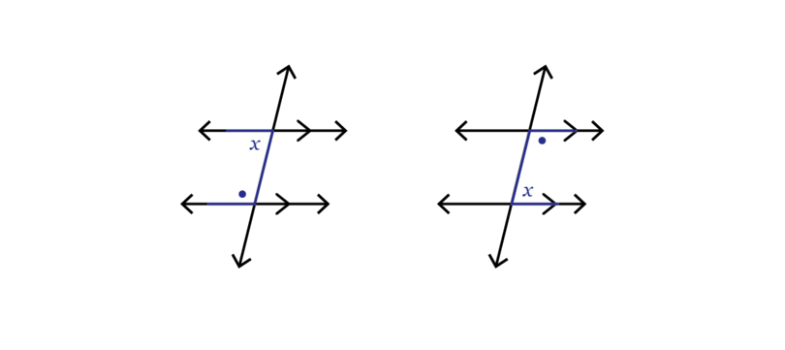Remember that alternate angles and corresponding angles are equal, but only if the lines cut by the transversal are parallel. Similarly, co-interior angles add up to $$180^\circ$$, only if the lines cut by the transversal are parallel.

## Worked example 12.7: Identifying angles on parallel lines

For the parallel lines intersected by a transversal shown in the diagrams below, identify:

1. one pair of corresponding angles
2. one pair of alternate angles
3. one pair of co-interior angles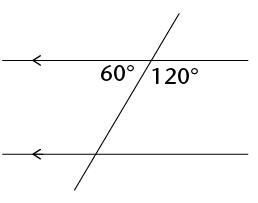### The angle corresponding to $$60^{\circ}$$ is shown in blue below. The two angles form a reflected F pattern. Remember that the two angles are equal because the lines forming the F are marked as being parallel.

Similarly, the angle corresponding to $$120^{\circ}$$ is shown in red. Notice that these angles form an F pattern.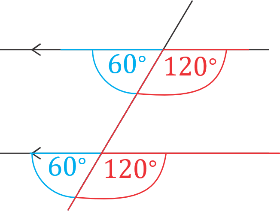### The angle alternate to $$60^{\circ}$$ is shown in blue below. The two angles form a Z pattern. Remember that the two angles are equal because it is given that the lines forming the Z are parallel.

Similarly, the angle alternate to $$120^{\circ}$$ is shown in red. Notice that the angles form a reflected Z pattern on alternate sides of the transversal.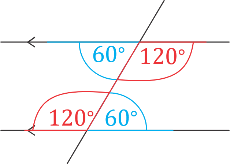### The angle co-interior to $$60^{\circ}$$ is shown in blue below. The two angles form a reflected C pattern. Remember that the two angles are supplementary because it is given that the lines forming the C are parallel ($$180^{\circ} - 60^{\circ} = 120^{\circ}$$).

Similarly, the other pair of supplementary co-interior angles are shown in red. Notice that the angles $$60^{\circ}$$ and $$120^{\circ}$$ form a C pattern on the same side of the transversal.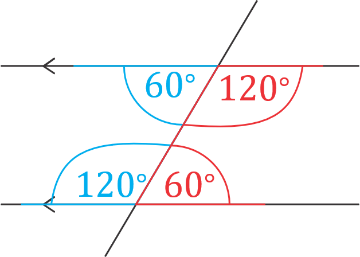temp text